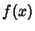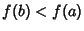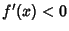## Decreasing Function

A functiondecreases on an Intervaliffor all, where. Conversely, a functionincreases on an Intervaliffor allwith.

If the Derivativeof a Continuous Functionsatisfieson an Open Interval, thenis decreasing on. However, a function may decrease on an interval without having a derivative defined at all points. For example, the functionis decreasing everywhere, including the origin, despite the fact that the Derivative is not defined at that point.